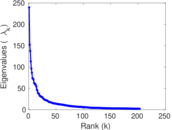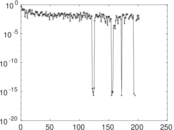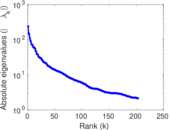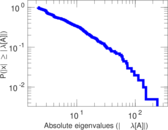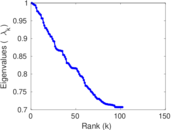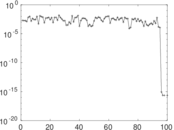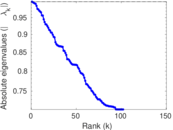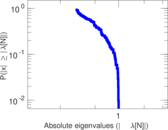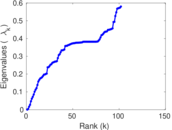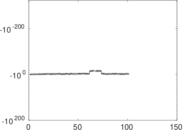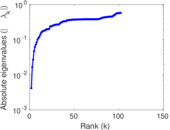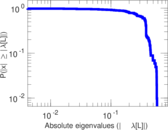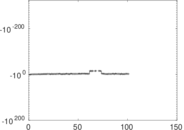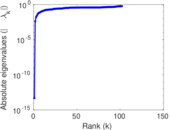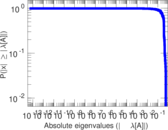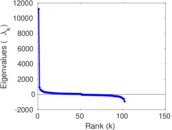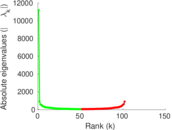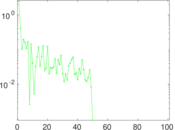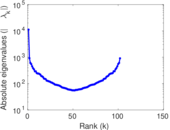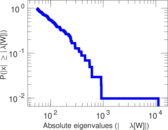# Wikipedia edits (lbe)

This is the bipartite edit network of the лакку Wikipedia. It contains users and pages from the лакку Wikipedia, connected by edit events. Each edge represents an edit. The dataset includes the timestamp of each edit.

 Code `lbe` Internal name `edit-lbewiki` Name Wikipedia edits (lbe) Data source http://dumps.wikimedia.org/ AvailabilityDataset is available for download Consistency checkDataset passed all tests Category Authorship network Dataset timestamp 2017-10-20 Node meaning User, article Edge meaning Edit Network formatBipartite, undirected Edge typeUnweighted, multiple edges Temporal dataEdges are annotated with timestamps

## Statistics

 Size n = 11,212 Left size n1 = 657 Right size n2 = 10,555 Volume m = 39,548 Unique edge count m̿ = 23,854 Wedge count s = 19,120,036 Claw count z = 20,047,198,159 Cross count x = 18,887,520,262,173 Square count q = 9,452,961 4-Tour count T4 = 152,164,488 Maximum degree dmax = 4,554 Maximum left degree d1max = 4,554 Maximum right degree d2max = 252 Average degree d = 7.054 58 Average left degree d1 = 60.194 8 Average right degree d2 = 3.746 85 Fill p = 0.003 439 83 Average edge multiplicity m̃ = 1.657 92 Size of LCC N = 10,646 Diameter δ = 13 50-Percentile effective diameter δ0.5 = 3.422 61 90-Percentile effective diameter δ0.9 = 5.174 20 Median distance δM = 4 Mean distance δm = 3.828 13 Gini coefficient G = 0.823 504 Balanced inequality ratio P = 0.139 704 Left balanced inequality ratio P1 = 0.079 523 6 Right balanced inequality ratio P2 = 0.209 088 Relative edge distribution entropy Her = 0.730 445 Power law exponent γ = 3.811 70 Tail power law exponent γt = 1.821 00 Tail power law exponent with p γ3 = 1.821 00 p-value p = 0.028 000 0 Left tail power law exponent with p γ3,1 = 1.691 00 Left p-value p1 = 0.000 00 Right tail power law exponent with p γ3,2 = 5.511 00 Right p-value p2 = 0.875 000 Degree assortativity ρ = −0.588 975 Degree assortativity p-value pρ = 0.000 00 Spectral norm α = 239.532 Algebraic connectivity a = 0.004 157 99 Spectral separation |λ1[A] / λ2[A]| = 1.572 16 Controllability C = 9,976 Relative controllability Cr = 0.891 351

## Plots

### Fruchterman–Reingold graph drawing### Degree distribution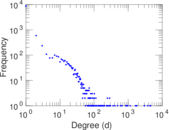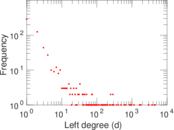### Cumulative degree distribution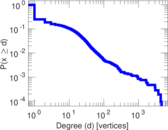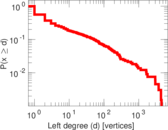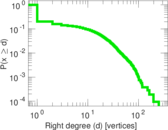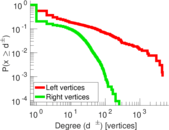### Lorenz curve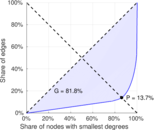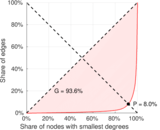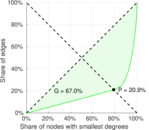### Spectral distribution of the adjacency matrix### Spectral distribution of the normalized adjacency matrix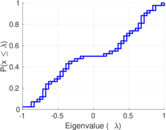### Spectral distribution of the Laplacian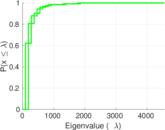### Spectral graph drawing based on the adjacency matrix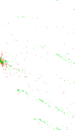### Spectral graph drawing based on the Laplacian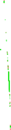### Spectral graph drawing based on the normalized adjacency matrix### Degree assortativity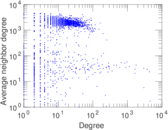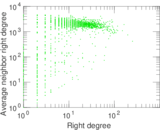### Zipf plot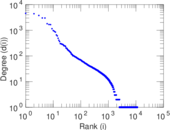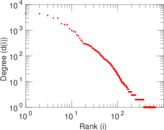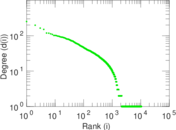### Hop distribution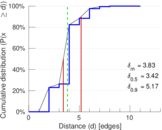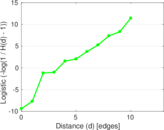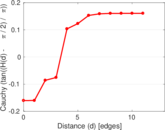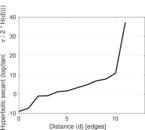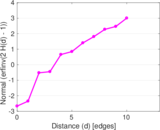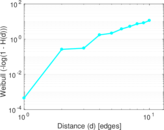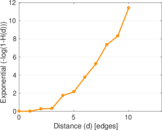### Double Laplacian graph drawing### Delaunay graph drawing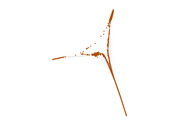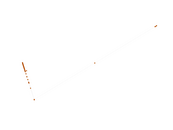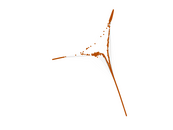### Edge weight/multiplicity distribution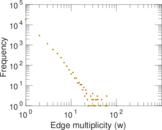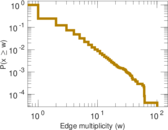### Temporal distribution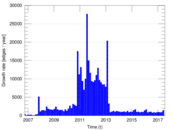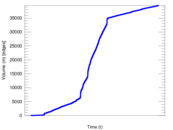### Temporal hop distribution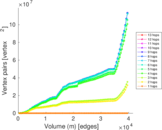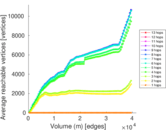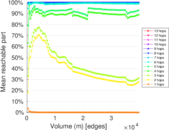### Diameter/density evolution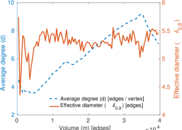### Matrix decompositions plots